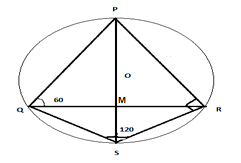Question 24

# P, Q, S, and R are points on the circumference of a circle of radius r, such that PQR is an equilateral triangle and PS is a diameter of the circle. What is the perimeter of the quadrilateral PQSR?

SolutionLet PQR be an equilateral triangle with side equal to x and let the intersection point of PS and QR be M.

Clearly, the circle is the circumcircle of the triangle PQR.

QR = x => QM = $$\frac{x}{2}$$ because a perpendicular from the centre to any chord bisects the chord.

Angle OQM = 30 degrees and QM is equal to $$\frac{x}{2}$$ => OQ = $$\frac{\frac{x}{2}}{cos(30)}$$ = $$\frac{x}{\sqrt{3}}$$

Hence the radius of the circumcircle of an equilateral triangle is equal to $$\frac{x}{\sqrt{3}}$$.

Angle PQS = 90 degrees as it is an angle in a semicircle. PS bisects angle QPR => angle QPS is 30 degrees. Hence QS subtends an angle of 30 degrees in the major arc => QS subtends an angle of 60 degrees at the centre because angle subtended by a chord at the centre is twice the angle subtended by the chord in the major arc.

Angle QOS = 60 degrees => Triangle QOS is equilateral and hence QS is equal to radius of the circle => QS = $$\frac{x}{\sqrt{3}}$$

Given that radius is r => r = $$\frac{x}{\sqrt{3}}$$ => x = $$r\sqrt{3}$$

=> Perimeter of PQRS = PQ+QS+SR+RP= $$r\sqrt{3} + r + r + r\sqrt{3}$$ = $$2r(1+\sqrt{3})$$